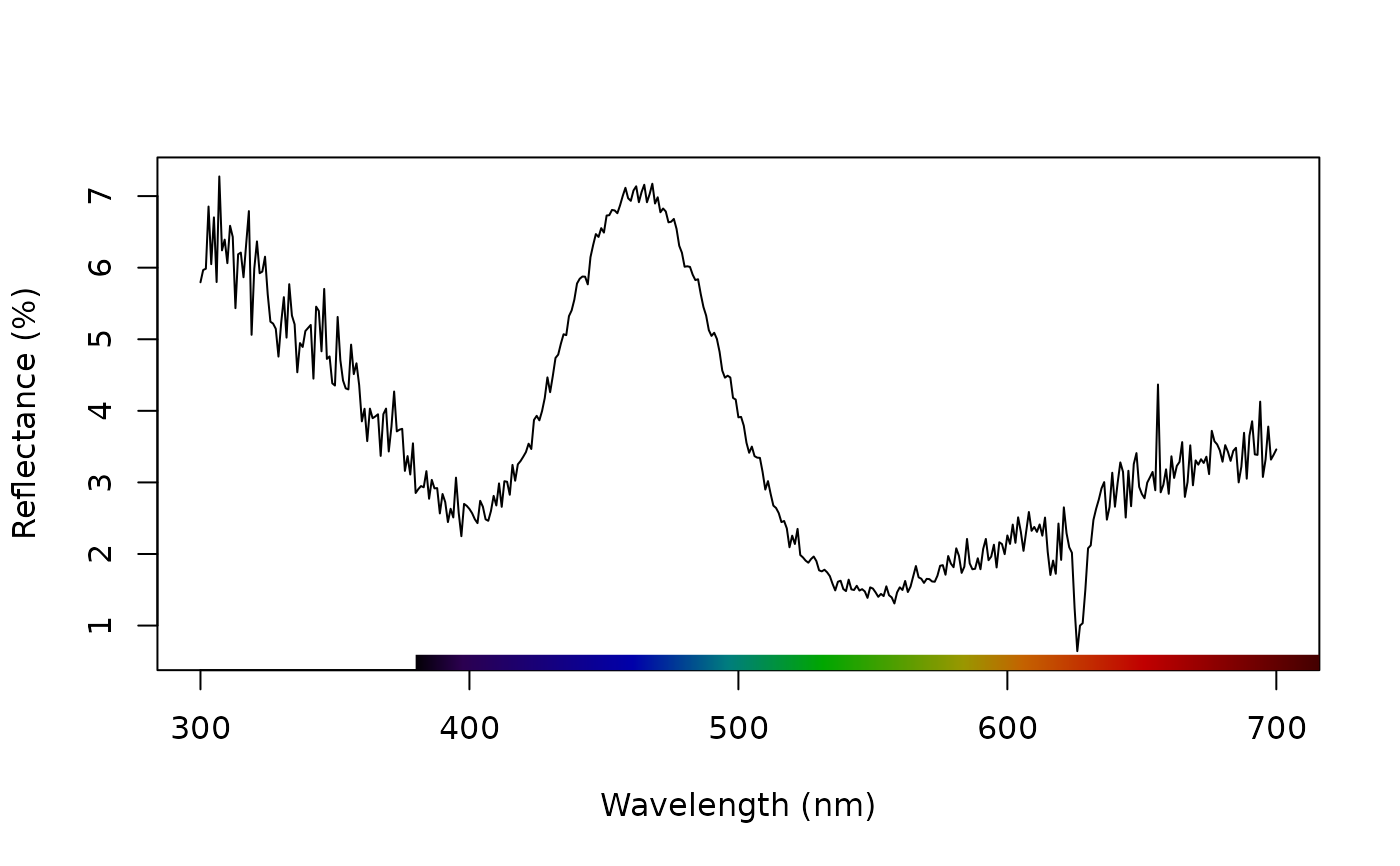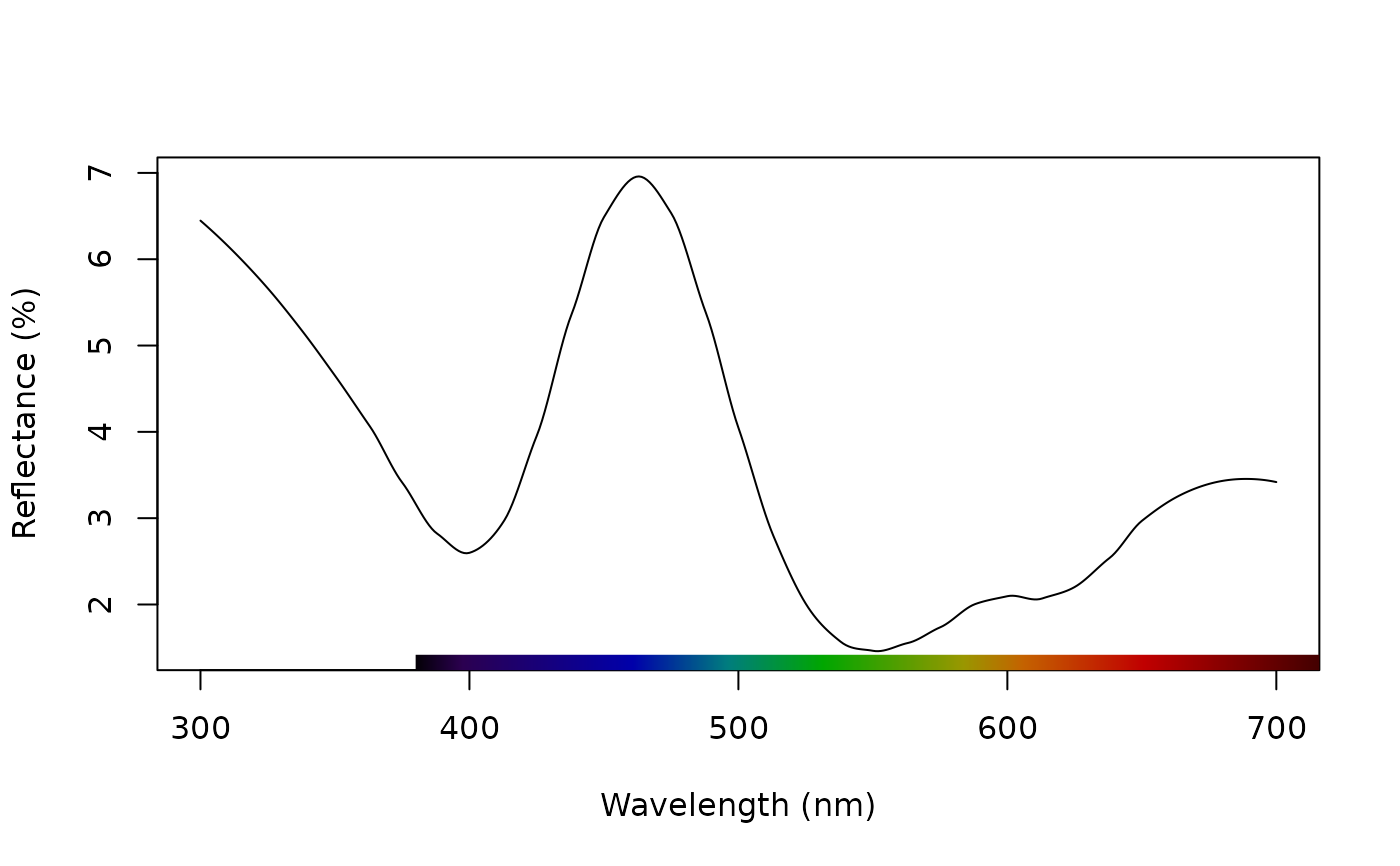Applies normalization and/or smoothing to spectra for further analysis or plotting.

procspec(
rspecdata,
opt = c("none", "smooth", "maximum", "minimum", "bin", "sum", "center"),
span = 0.25,
bins = 20
)

## Arguments

rspecdata (required) a data frame, possibly of class rspec, which contains a column containing a wavelength range, named 'wl', and spectra data in remaining columns. what type of processing options to apply. User can select multiple options by providing a vector. Possibilities are: "none" does not perform any processing (default). "smooth" applies LOESS smoothing to each spectrum using loess.smooth(). Optimal smoothing parameter can be assessed by using plotsmooth(). "minimum" subtracts the minimum from each individual spectra. "maximum" divides each spectrum by its maximum value. "sum" divides each spectrum by summed values. "bin" bins each spectrum into the specified number of bins. bins argument must be set. "center" centers individual spectra by subtracting mean reflectance from all values. how to handle negative values. Possibilities are: "none" does not perform negative value correction (default). "zero" sets all negative values to zero. "addmin" adds the absolute value of the maximally negative values of each spectra to the reflectance at all other wavelengths (setting the minimum value to zero, but scaling other values accordingly). sets the smoothing parameter used by loess.smooth(). sets the number of equally sized wavelength bins for opt = "bin".

## Value

A data frame of class rspec with the processed data.

Cuthill, I., Bennett, A. T. D., Partridge, J. & Maier, E. 1999. Plumage reflectance and the objective assessment of avian sexual dichromatism. The American Naturalist, 153, 183-200.

Montgomerie R. 2006. Analyzing colors. In Hill, G.E, and McGraw, K.J., eds. Bird Coloration. Volume 1 Mechanisms and measurements. Harvard University Press, Cambridge, Massachusetts.

White, T. E., Dalrymple, R. L., Noble D. W. A., O'Hanlon, J. C., Zurek, D. B., Umbers, K. D. L. 2015. Reproducible research in the study of biological coloration. Animal Behaviour, 106, 51-57.

loess.smooth(), plotsmooth()

## Examples

data(teal)
plot(teal, select = 10)# Smooth data to remove noise
teal.sm <- procspec(teal, opt = "smooth", span = 0.25)
#> processing options applied:
#> smoothing spectra with a span of 0.25plot(teal.sm, select = 10)# Normalize to max of unity
teal.max <- procspec(teal, opt = c("max"))
#> processing options applied:
#> Scaling spectra to a maximum value of 1plot(teal.max, select = 10)